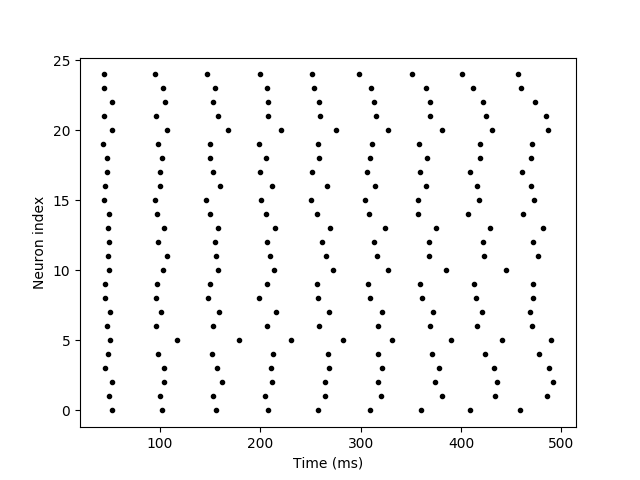# Example: non_reliability¶

Note

You can launch an interactive, editable version of this example without installing any local files using the Binder service (although note that at some times this may be slow or fail to open):Reliability of spike timing.

See e.g. Mainen & Sejnowski (1995) for experimental results in vitro.

Here: a constant current is injected in all trials.

from brian2 import *

N = 25
tau = 20*ms
sigma = .015
eqs_neurons = '''
dx/dt = (1.1 - x) / tau + sigma * (2 / tau)**.5 * xi : 1 (unless refractory)
'''
neurons = NeuronGroup(N, model=eqs_neurons, threshold='x > 1', reset='x = 0',
refractory=5*ms, method='euler')
spikes = SpikeMonitor(neurons)

run(500*ms)
plot(spikes.t/ms, spikes.i, '.k')
xlabel('Time (ms)')
ylabel('Neuron index')
show()